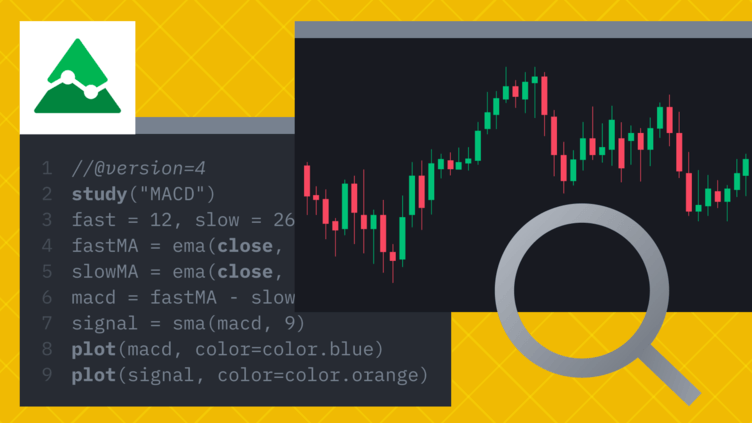HomeArticles

# How To Create TA Indicators on TradingView

1mo ago
12m

Contents

## Introduction

Without the right trading tools, you can’t conduct effective technical analysis. A strong trading strategy will help you avoid common mistakes, improve your risk management, and increase your ability to identify and take advantage of opportunities.

For many, TradingView is the go-to charting platform. Offering a hub of technical analysis tools, the powerful HTML5 web application is used by millions to track movements in the Forexcryptocurrency, and traditional stock markets.

TradingView has many powerful features: it allows us to track assets across numerous trading platforms and to publish trading ideas within its social network. In this article, we’ll focus on its customizability. We’ll be using Pine Script, TradingView’s own programming language, which grants us granular control over our chart layouts.

Let’s get started!

## What is Pine Script?

Pine Script is a scripting language that can be used to modify your TradingView charts. The platform already equips you with many features to do so, but Pine Script allows you to take it a step further. Whether you want to change the colors of your candlesticks or to backtest a new strategy, the Pine Editor will enable you to customize your real-time charts as you see fit.

The code itself is excellently documented, so be sure to check out the user manual for more information. Our goal in this tutorial is to discuss some of the basics and introduce indicators that can come in handy for cryptocurrency trading.

## Setting up

It’s incredibly simple to get started with Pine Script. Any code we write is run on TradingView’s servers, so we can access the editor and develop our scripts from a browser – without any additional download or configuration required.

In this tutorial, we’re going to chart the Bitcoin/Binance USD (BTCBUSD) currency pair. If you don’t have one already, go ahead and create a free account (there’s a pro subscription available, too, but it isn’t necessary for this guide).

Follow this link, and you’ll see a chart resembling the following: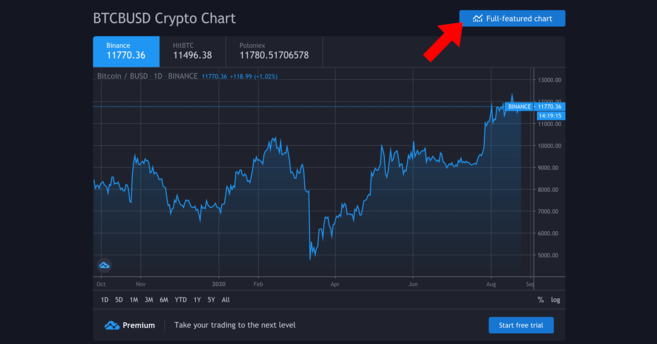Yours will probably be more up to date.

Here, we want to get the full-featured chart – click on the button to access it. That gives us a much more detailed view, drawing tools, and options to plot trendlines, amongst other things.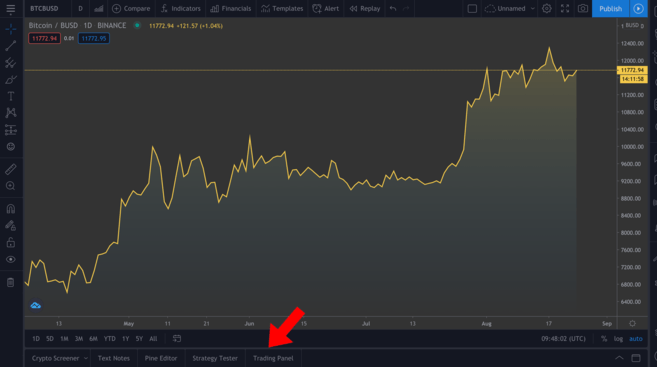The full-featured chart. You can adjust the timeframe by clicking on the views above the highlighted tabs.

We won’t be discussing how to use the various tools available, but if you’re serious about technical analysis, we highly advise that you familiarize yourself with them. On the bottom left (outlined in the image), you’ll see a few different tabs – click on Pine Editor.

## The Pine Editor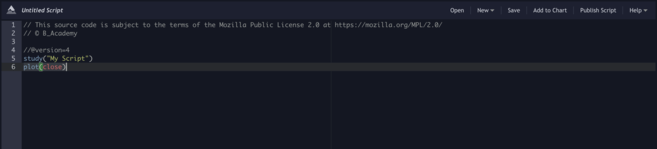This editor is where the magic happens. We’ll tell it what we want to do, then click on Add to Chart to see our annotations appear above. Note that things can get messy if we include several annotations at once, so we’ll remove them between examples (right-click in the chart > Remove Indicators).

You can see that we already have a couple of lines of code there. Let’s click Add to Chart to see what happens.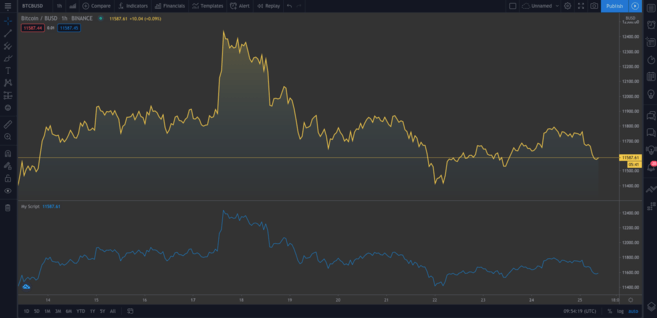A second chart gets added beneath the original. The new chart happens to represent the same data. Hover over My Script and click on the cross to remove it. Now, let’s dig into the code.

``study("My Script")``

This first line is just setting up our annotation. It only requires the name that you want to call the indicator ("My Script", in this case), but there are also some optional parameters we can add. One of those is overlay, which tells TradingView to place the indicator on the existing chart (instead of in a new segment). As you can see from our first example, it defaults to false. Though we won’t see it in action now, overlay=true adds the indicator to the existing chart.

``plot(close)``

This line is the instruction to plot the close price of Bitcoin. plot simply gives us a line chart, but we can also display candlesticks and bars, as we’ll see shortly.

Now, let’s try the following:

``````//@version=4
study("My Script", overlay=true)
plot(open, color=color.purple)``````

Once you add this, you should see a second chart (which looks like the original shifted to the right). All we’ve done is plotted the open price instead, and since the current day’s open is the previous day’s close, it makes sense that these have an identical shape.

Okay! Let’s get rid of the current annotations (remember, we do that by right-clicking and hitting Remove Indicators). Hover over Bitcoin / BUSD and click the Hide button to clear the current chart, too.

Many traders prefer candlestick charts as they give us more information than a simple plot like the one we just did. Let’s add them next.

``````//@version=4
study("My Script", overlay=true)
plotcandle(open, high, low, close)``````

It’s a good start, but the lack of colors makes it a bit bland. Ideally, we should have red candles when the open is greater than the close for the given timeframe, and green ones if the close exceeds the open. We’ll add a line above the plotcandle() function:

``````//@version=4
study("My Script", overlay=true)
colors = open >= close ? color.red : color.green
plotcandle(open, high, low, close)``````

This looks at every candlestick and checks whether the open is greater or equal to the close. If it is, it means prices have dropped over the period, so it will color the candlestick red. Otherwise, it will color it green. Modify the plotcandle() function to pass this color scheme in:

``````//@version=4
study("My Script", overlay=true)
colors = open >= close ? color.red : color.green
plotcandle(open, high, low, close, color=colors)``````

Remove the current indicators if you haven’t already, and add this one to the chart. Now we should have something resembling a regular candlestick chart.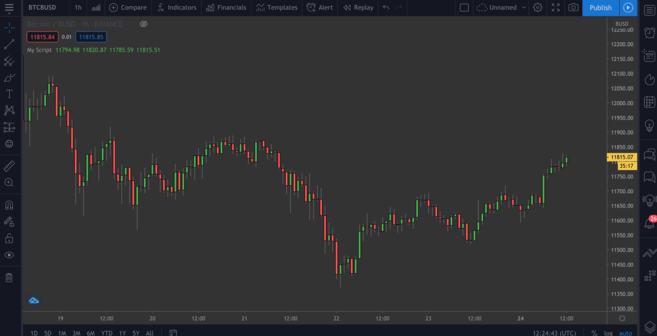Beautiful!

## Plotting moving averages (MA)

We’ve got some of the basics down. Let’s move on to our first custom indicator – the exponential moving average, or EMA. This is a valuable tool as it allows us to filter out any market noise and smooth out price action.

EMA differs slightly from the simple moving average (SMA), in that it puts more weight in the most recent data. It tends to be more reactive to sudden movements and is often used for short-term plays (in day trading, for example).

### The simple moving average (SMA)

We might as well plot the SMA, just so we can compare the two after. Add this line to your script:

``plot(sma(close, 10))``

This plots the average of the previous ten days. Tweak the number in the brackets to see how the curve changes when taking into account different lengths.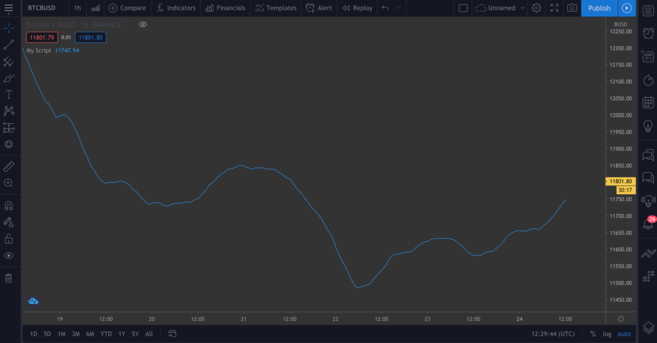The SMA, based on the previous ten days.

### The exponential moving average (EMA)

The EMA will be a bit trickier to understand, but not to worry. Let’s break down the formula first:

``EMA = (Close - Previous Day’s EMA) * Multiplier - Previous Day’s EMA``

So, what’s this telling us? Well, for each day, we calculate a new moving average based on the previous day’s one. The multiplier is what “weighs” the most recent period, and is calculated with the following formula:

``Multiplier = 2 / (Length of EMA + 1)``

As with simple moving averages, we need to specify how long the EMA will be. Syntactically, the function to plot EMA is similar to the SMA one. Plot it alongside the SMA so you can compare the two:

``````//@version=4
study("My Script", overlay=true)
plot(sma(close, 10))
plot(ema(close,10))``````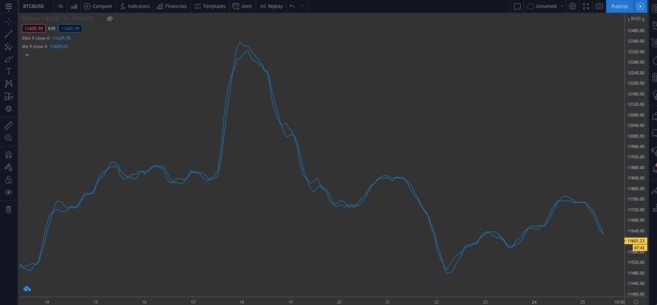You can see a slight difference in the two types of MA.

### Built-in scripts

So far, we’ve written our code manually so you can get a feel for it. But let’s introduce something that can save us time, particularly if we’re writing more complex scripts, and we don’t want to do them from scratch.

On the top, right-hand side of your editor, click on New. You’ll get a dropdown menu with all kinds of different technical indicators. Click Moving Average Exponential to see the source code for an EMA indicator.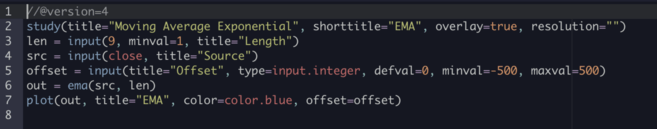This one is different from ours – you’ll notice the input() functions. These are nice from a usability perspective since you can click on this box…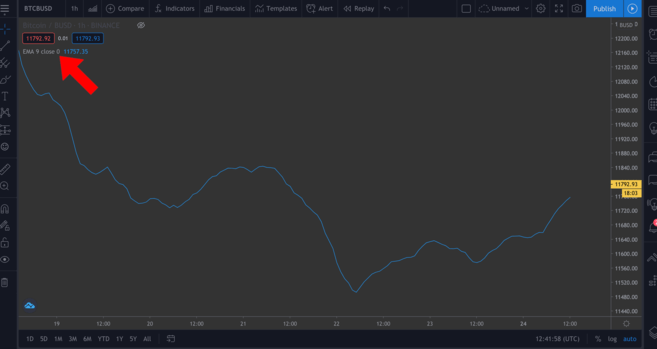...and easily change some of the values in a pop-up window by clicking on the Settings wheel.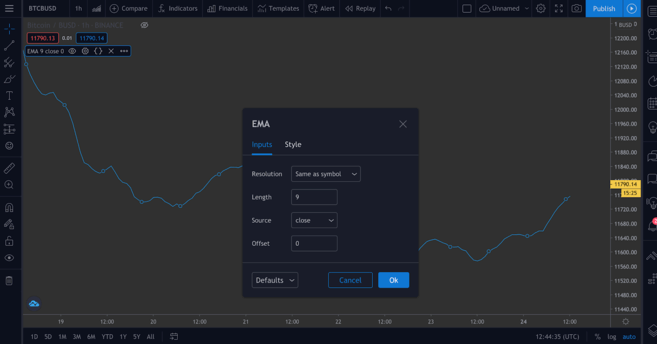We’ll add a couple of input() functions in our next script to demonstrate this.

## Plotting the Relative Strength Index (RSI) indicator

The Relative Strength Index (RSI) is another essential indicator in technical analysis. It’s known as a momentum indicator, meaning that it measures the rate at which assets are bought and sold. Presented on a scale of 0 to 100, an RSI score attempts to inform investors on whether assets are overbought or oversold. Typically, an asset may be considered oversold if it has a score less than or equal to 30, and it could be overbought with a score greater or equal to 70.

If you head to New > RSI Strategy, you can see this for yourself. RSI is generally measured over periods of 14 (i.e., 14 hours or 14 days), but you’re free to tweak that setting to suit your own strategy.

Add this to the chart. You should see a few arrows displayed now (defined by the strategy.entry() function in the code). RsiLE indicates a potential opportunity to long the asset as it may be oversold. RsiSE highlights possible points at which to short the asset when it’s overbought. Note that, as with all indicators, you shouldn’t necessarily rely on these as foolproof evidence that prices will decrease/increase.

## Backtesting

There is a way for us to test our custom indicators. Though past performance is no guarantee of future results, backtesting our scripts can give us an idea of how effective they are at picking up signals.

We’ll give an example of a simple script below. We’re going to create a straightforward strategy that enters a long position when the BTC price falls below \$11,000 and exits the position when the price exceeds \$11,300. We can then see how profitable this strategy would have been historically.

``````//@version=4
strategy("ToDaMoon", overlay=true)
enter = input(11000)
exit = input(11300)
price = close

if (price <= enter)
if (price >= exit)
strategy.close_all(comment="SellTheNews")``````

Here we’ve defined entry and exit as variables – both are inputs, meaning that we can change them on the chart later. We also set up the price variable, which takes the close for each period. Then, we have some logic in the form of if statements. If the part in brackets is true, then the indented block beneath it will be run. Otherwise, it will be skipped.

So, if the price is less than or equal to our desired entry, the first expression evaluates as true, and we’ll open a long position. Once the price equals or exceeds the desired exit, the second block will be triggered, closing all open positions.

We’ll annotate the chart with arrows that show where we’ve entered/exited, so we’ve specified what to label these points with the comment parameter (in this example, “BuyTheDip” and “SellTheNews”). Copy the code, and add it to the chart.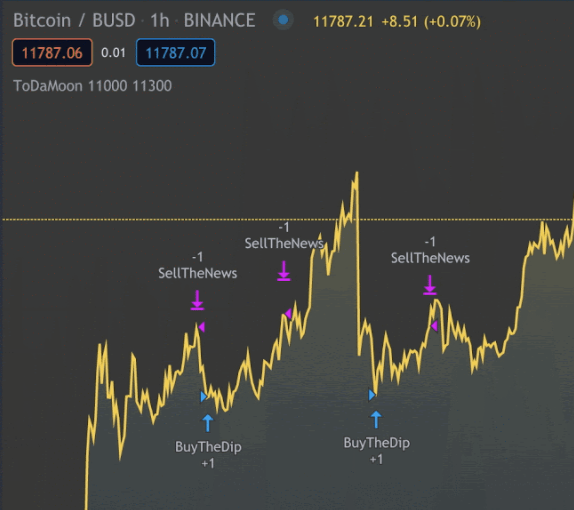You can now see the indicators on the chart. You might need to zoom out.

TradingView automatically applies your rules to older data. You’ll also notice that it switches from the Pine Editor to the Strategy Tester tab. This allows you to see an overview of your potential profits, a list of trades, and each of their individual performances.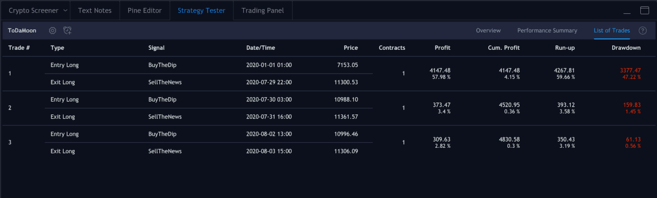Positions we’ve entered and exited.

## Tying it together

Time to write our own script by using some of the concepts we’ve seen so far. We’re going to combine EMA and RSI and use their values to color candlesticks, yielding insights that we can easily visualize.

This shouldn’t be construed as financial advice – there’s no objectively correct way to use this indicator. As with all others, it should be used with other tools to develop your own strategy.

Now let’s work on our new script. Remove all your indicators from the chart, and hide the Bitcoin/BUSD chart, too, so that we have a clean canvas to work on.

Let’s start by defining our study. Feel free to name it whatever you want, just make sure to set overlay=true.

``study(title="Binance Academy Script", overlay=true)``

Remember our EMA formula from earlier. We need to provide the multiplier with the length of the EMA. Let’s make it an input that requires an integer (so, no decimal places). We’ll also set a minimum that it can be (minval), and a default value (defval).

``````study(title="Binance Academy Script", overlay=true)
emaLength = input(title="EMA Length", type=input.integer, defval=25, minval=0)``````

Using this new variable, we can calculate the EMA value for each candle in our chart:

``````study(title="Binance Academy Script", overlay=true)
emaLength = input(title="EMA Length", type=input.integer, defval=25, minval=0)
emaVal = ema(close, emaLength)``````

Great. Onto the RSI. We’ll give it a length in a similar way:

``````study(title="Binance Academy Script", overlay=true)
emaLength = input(title="EMA Length", type=input.integer, defval=25, minval=0)
emaVal = ema(close, emaLength)
rsiLength = input(title="RSI Length", type=input.integer, defval=25, minval=0)``````

And now, we can calculate it:

``````study(title="Binance Academy Script", overlay=true)
emaLength = input(title="EMA Length", type=input.integer, defval=25, minval=0)
emaVal = ema(close, emaLength)
rsiLength = input(title="RSI Length", type=input.integer, defval=25, minval=0)
rsiVal = rsi(close, rsiLength)``````

At this stage, let’s put together the logic that colors the candlesticks depending on the EMA and RSI values. Let’s take a situation where (a) the close price of the candle exceeds the EMA and (b) where the RSI is above 50.

Why? Well, you might decide that these indicators can be used in conjunction to tell you when to long or short Bitcoin. For instance, you might think that satisfying both of these conditions means that it’s a good time to enter a long position. Or conversely, you might use it to inform you when not to short, even if other indicators say otherwise.

So, our next line will look like this:

``````study(title="Binance Academy Script", overlay=true)
emaLength = input(title="EMA Length", type=input.integer, defval=25, minval=0)
emaVal = ema(close, emaLength)
rsiLength = input(title="RSI Length", type=input.integer, defval=25, minval=0)
rsiVal = rsi(close, rsiLength)
colors = close > emaVal and rsiVal > 50 ? color.green : color.red``````

If we translate this into plain English, we’re merely saying that if the EMA value exceeds the close price and the RSI score exceeds 50, we’ll color the candle green. Otherwise, we’ll color it red.

Next, plot the EMA:

``````study(title="Binance Academy Script", overlay=true)
emaLength = input(title="EMA Length", type=input.integer, defval=25, minval=0)
emaVal = ema(close, emaLength)
rsiLength = input(title="RSI Length", type=input.integer, defval=25, minval=0)
rsiVal = rsi(close, rsiLength)
colors = close > emaVal and rsiVal > 50 ? color.green : color.red
plot(emaVal, "EMA")``````

Lastly, plot the candles, making sure to include the color parameter:

``````study(title="Binance Academy Script", overlay=true)
emaLength = input(title="EMA Length", type=input.integer, defval=25, minval=0)
emaVal = ema(close, emaLength)
rsiLength = input(title="RSI Length", type=input.integer, defval=25, minval=0)
rsiVal = rsi(close, rsiLength)
colors = close > emaVal and rsiVal > 50 ? color.green : color.red
plot(emaVal, "EMA")
plotcandle(open, high, low, close, color=colors)``````

And that’s the script! Add it to the chart to see it in action.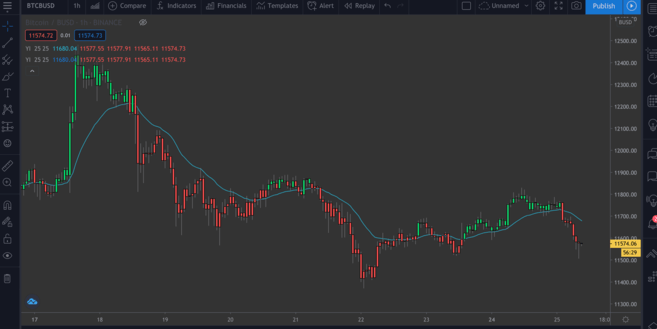A BTC/BUSD chart with the EMA/RSI indicator.

## Closing thoughts

In this article, we’ve run through some basic examples of what you can do with TradingView’s Pine Editor. By now, you should be confident in making simple annotations to price charts to gain additional insights from your own indicators.

We’ve only taken an interest in a couple of indicators here, but it’s easy to spin up more complex ones – either by selecting the inbuilt scripts from New or by writing them yourself.

Lacking inspiration? The following articles might provide you with some ideas for your next project: